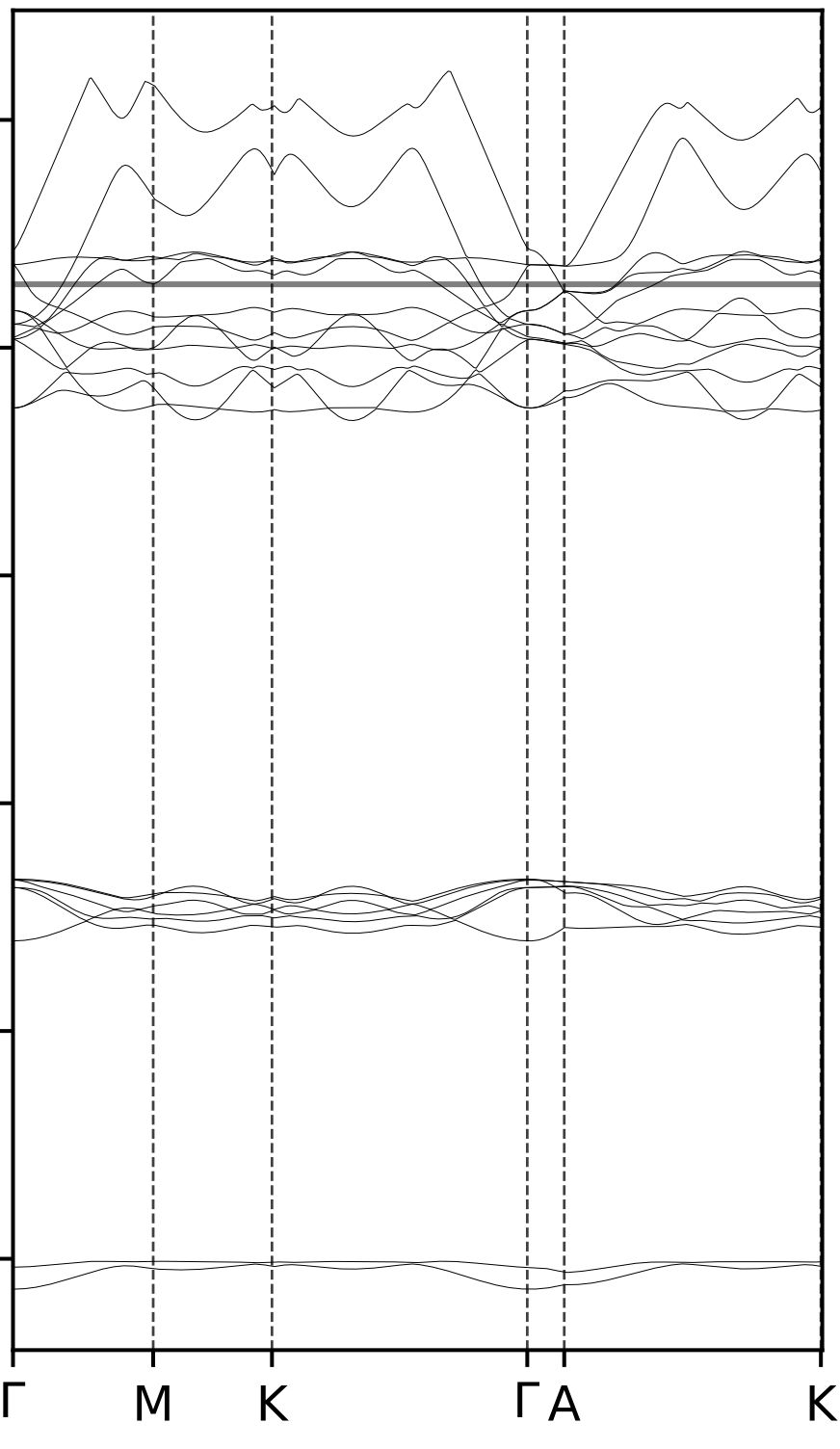# Band Diagram on Quantum ESPRESSO

Posted by Jingyi on January 28, 2019

Thanks for the Band Diagram Tutorial for Quantum ESPRESSO

Dependence

1. Quantum Espresso

2. Python3 + Numpy + Matplotlib

Workflow of band diagram

graph TD; A["pw.x: vc-relax"]-->F["pw.x: scf"]; F-->B["pw.x: nscf"]; A-- or -->B; B-->C["pw.x: bands"]; C-->D["bands.x"]; D-. matplotlib .->E["band diagram"]

The procedures in this workflow should use the same outdir and prefix configurations.

# pw.x

pw.x input descirption

&control and etc. settings for each procedure:

## vc-relax

1
2
&control
calculation='vc-relax',


## scf

1
2
&control
calculation='scf',


## nscf

1
2
&control
calculation='nscf',


## bands

1
2
&control
calculation='bands',


after CELL_PARAMETERS section, involve

1
2
3
4
5
K_POINTS crystal
k_points_in_total
a   b   c   wk
a   b   c   wk
a   b   c   wk


The K_POINTS here should follow certain K-path.

The k points settings share the same file coord.txt as in How to Generate QHA Input.

# bands.x

bands.x input description

The input for bands.x would be like

1
2
3
4
5
&BANDS
prefix="the_prefix"
outdir="the_outdir"
filband="Band.dat"
/


# Plot band diagram

Learn about the Quantum ESPRESSO output from bands.x

There are several output types (supposed using filband="Band.dat" in the input for bands.x):

1. Band.dat.gnu with bands in eV, directly plottable using gnuplot

2. Band.dat.rap with symmetry information, to be read by plotting code plotband.x

3. Band.dat containing the band structure, in a format suitable for plotting code plotband.x

4. bandx.out

5. scf.out or nscf.out Provide the Fermi Energy.

Here, we are using Band.dat to provide band energy, K_Path, and high-symmetry information, scf.out to provide Fermi Energy.

1
2
3
4
5
6
7
8
9
10
11
12
13
14
15
16
17
18
19
20
21
22
23
24
25
26
27
28
29
30
31
32
33
34
35
36
37
38
39
40
41
42
43
44
45
46
47
48
49
50
51
52
53
54
55
56
57
import numpy as np
import matplotlib.pyplot as plt
import os
import re

def dist(a,b):
# calculate the distance between vector a and b
d = ((a-b) ** 2 + (a-b) ** 2 + (a-b) ** 2)**0.5
return d

# Read the fermi energy in scf.out
fermi = 0
with open(file_name, "r") as f:
for line in lines:
if "the Fermi energy" in line:
fermi = float(line.split())

return fermi

# Read the bands in Band.dat
coord_regex = r"^\s+(.\d+.\d+)\s+(.\d+.\d+)\s+(.\d+.\d+)\$"
x_coord = []
x = []
bands = dict()

with open(file_name, "r") as f:

for i in range(len(lines)):
line = lines[i]
match = re.match(coord_regex,line)
if match:
x_coord.append([float(match.group(1)), float(match.group(2)), float(match.group(3)) ])
bandddd = lines[i+1] + lines[i+2]
bandddd = bandddd.split()

for j in range(len(bandddd)):
if j not in bands.keys():
bands[j] = []
bands[j].append(float(bandddd[j]))
for i in range(len(x_coord)) :
if i == 0:
x.append(0)
else:
x.append( x[-1] + dist(x_coord[i], x_coord[i-1]))
return bands,x

def plot(bands, x, fermi):
xaxis = [min(x),max(x)]
for i in bands.values():
plt.plot(x, i, color=color_dic[pressure], lw=0.2)
plt.plot(xaxis, [fermi, fermi], color="#66ccff",ls="solid", alpha = 0.5,lw = 1.2)
plt.xlim(xaxis)
`
• Plot example: